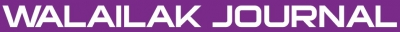A New Efficient Algorithm to Solve Non-Linear Fractional Ito Coupled System and Its Approximate Solution

Sunil KUMAR

Abstract

The aim of the present article is to obtain the approximate analytical solution of time fractional Ito coupled equations by using the homotopy perturbation method. The fractional derivatives are described in the Caputo sense. The highlight of the paper is error analysis between the exact solutions and approximate solutions which shows that our approximate solutions converge very rapidly to the exact solutions. The method gives analytical solution in the form of a convergent series with easily computable components, requiring no linearization or small perturbation. The results reveal that the proposed method is very effective and simple.

doi:10.14456/WJST.2014.60

Keywords

Ito coupled system, fractional derivative, Caputo derivatives, approximate solution, fractional Brownian motion

PDF

References

KB Oldham and J Spanier. The Fractional Calculus. Academic Press, New York, 1974.

KS Miller and B Ross. An Introduction to the Fractional Calculus and Fractional Differential Equations. Johan Willey and Sons, New York, 2003.

I Podlubny. Fractional Differential Equations Calculus. Academic Press, New York, 1999.

AA Kilbas, HM Srivastava and JJ Trujillo. Theory and Applications of Fractional Differential Equations. Elsevier, Amsterdam, 2006.

I Podlubny. Geometric and physical interpretation of fractional integration and fractional differentiation. Fract. Calc. Appl. Anal. 2002; 5, 367-86.

JH He. Homotopy perturbation technique. Comput. Meth. Appl. Mech. Eng. 1999; 178, 257-62.

JH He. Application of homotopy perturbation method to nonlinear wave equations. Chaos Soliton. Fract. 2005; 26, 695-700.

JH He. A coupling method of a homotopy technique and a perturbation technique for non-linear problems. Int. J. Nonlinear Mech. 2000; 35, 37-43.

A Yildirim and H Kocak. Homotopy perturbation method for solving the space-time fractional Advection-dispersion equation. Adv. Water Resour. 2009; 32, 1711-6.

S Kumar, Y Khan and A Yildirim. A mathematical modelling arising in the chemical system and its approximate solution. Asia Pac. J. Chem. Eng. 2012; 7, 835-40.

S Kumar, H Kocak and A Yildirim. A fractional model of gas dynamics equation by using Laplace transform. Z. Naturforsch. 2012; 67, 389-96.

S Kumar, MP Tripathi and OP Singh. A fractional model of Harry Dym equation and its approximate solution. Ain Shams Eng. J. 2013; 4, 111-5.

S Kumar and OP Singh. Inversion of Abel integral equation by homotopy perturbation method. Z. Naturforsch. 2010; 65, 677-82.

Z Odibat and S Momani. Modified homotopy perturbation method: Application to quadratic Riccati differential equation of fractional order. Chaos Soliton. Fract. 2008; 36, 167-74.

Q Wang. Homotopy perturbation method for fractional K dV equation. Appl. Math. Comput. 2007; 190, 1795-802.

ZZ Ganji, DD Ganji and Y Rostamiyan. Solitary wave solutions for a time-fraction Generalized Hirota-Satsuma coupled KdV equation by an analytical technique. Appl. Math. Model. 2009; 33, 3107-13.

A Golbabai and K Sayevand. The homotopy perturbation method for multi-order time fractional differential equations. Nonlinear Sci. Lett. A 2010; 1, 147-54.

MM Rashidi, DD Ganji and S Dinarvand. Explicit analytical solutions of the generalized Burgers and Burgers-Fisher equations by homotopy perturbation method. Numer. Meth. Part. Differ. Equat. 2009; 25, 409-17.

MM Rashidi and DD Ganji. Homotopy perturbation combined with Pad’e approximation for solving two dimensional viscous flow in the extrusion process. Int. J. Nonlinear Sci. 2009; 7, 387-94.

Y Chen and H An. Homotopy perturbation method for a type of nonlinear coupled equations with parameters derivatives. Appl. Math. Comput. 2008; 204, 764-72.

D Slota. The application of the homotopy perturbation method to one phase inverse Stefan problem. Int. Comm. Heat Mass Tran. 2010; 37, 587-92.

HA Zedan. Symmetry analysis of an Integrable Ito coupled system. Comput. Math. Appl. 2010; 60, 3088-97.

AM Kawala. Numerical solutions for Ito coupled system. Acta Appl. Math. 2009; 106, 325-35.

HA Zedan and EA Aidrous. Numerical solutions for a generalized Ito system by using Adomian decomposition method. Int. J. Math. Comput. 2009; 4, 9-19.

X Zhao, H Zhi and H Zhang. Improved Jacobi function with symbolic computation to construct new double- periodic solutions for the generalized Ito system. Chaos Soliton. Fract. 2006; 28, 112-26.

QP Liu. Hamiltonian structure for Ito system. Phys. Lett. A 2000; 277, 31-4.

AM Samoilenko, OM Stanzhytskyj and AM Ateiwi. On invariant Tori for a Stochastic Ito system. J. Dynam. Differ. Equat. 2005; 17, 737-58.

A Karasu. Comment: On a recently proposed relation between oHS and Ito systems. Phys. Lett. A 1999; 259, 168-70.

Y Xu and CW Shu. Local discontinuous Glerkin methods for the Kuramoto-Shivashinsky equations and the Ito type coupled Kdv equations. Comput. Meth. Appl. Mech. Eng. 2006; 195, 3430-47.

KK Ayse, K Atalay and SY Sakovich. Integrability of a generalized Ito system: The Painleve test. J. Phys. Soc. Jpn. 2001; 70, 1165-6.

HW Tam, WX Ma, XB Hu and DL Wang. The Hirota-Satsuma coupled Kdv equation and a coupled Ito system revisited. J. Phys. Soc. Jpn. 2000; 69, 45-51.

Y Luchko and R Gorneflo. The Initial Value Problem for Some Fractional Differential Equations with the Caputo Derivatives. Fachbreich Mathematick und Informatik, Freic Universitat Berlin, Germany, 1998.

Refbacks

• There are currently no refbacks.Online ISSN: 2228-835X

http://wjst.wu.ac.th

Last updated: 20 June 2019